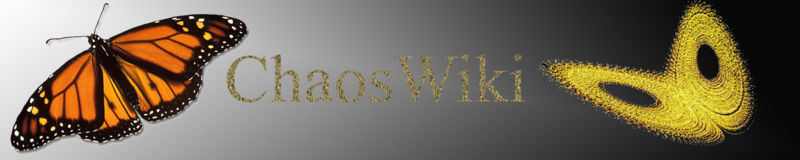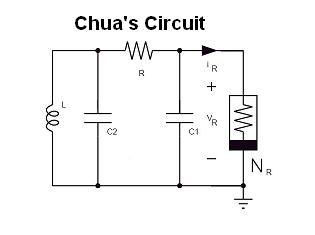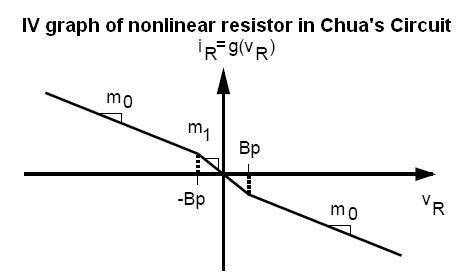Chaos is a fascinating nonlinear phenomenon. Dr. Leon Chua invented Chua's circuit (circa 1983), a simple nonlinear circuit capable of producing strange attractors. Before you can get started on Chua's circuit, it would be instructive to understand the basic concept of nonlinear circuits: the DP (or driving-point) plot. This term was coined in the classic book "Linear and Nonlinear Circuits" by Chua, Leon O., Desoer, Charles A. and Kuh, Ernest S. 1987. McGraw-Hill. ISBN 0070108986. UNFORTUNATELY THIS BOOK IS OUT OF PRINT. Here is the Amazon link to where you may find used copies of the book. But, here is a document on introductory nonlinear circuit analysis that I wrote for sophomore electronics students. This should be enough background for you to understand the implementation of Chua's circuit.

Once you understand the basics of nonlinear circuit analysis, here are some links and papers to get you started with chaos and Chua's circuit:
1. What is Chaos?
2. The Chaos hypertextbook
3. Michael Peter Kennedy's classic op-amp implementation of Chua's circuit

First we need to simulate Chua's circuit. The simulation tool we use is MultiSim. Here is the MultiSim schematic, click for a screen shot. Two points: First, this version of the circuit uses the LMC6482 which is more robust and easier to obtain than the JFET op-amps used in Michael Peter Kennedy's paper. A more subtle point is the series resistance of the inductor. YOU HAVE TO TAKE THIS INTO ACCOUNT WHILE BUILDING CHUA'S CIRCUIT.

Here I provide links and datasheets to the two most important items in the circuit: the dual op-amp and inductor.
• LMC6482AIN, Datasheet. Cost: \$2.29.
• API Devlan 18 mH inductor (digikey part number DN7452-ND). Note: This part is pretty difficult to get.
The rest of the components in the circuit are standard: a 2k pot, a 100 nF capacitor, a 10 nF capacitor and 22k x 2, 220 ohm x 2, 2.2k, 3.3k resistors and +/- 9 V batteries. You can find these at a local Radioshack. Hence, the total cost of the circuit is around \$10!

• First, you need a way to look at the waveforms from Chua's circuit. Osoop is open-source software that converts your computer into an oscilloscope by using your soundcard as an analog-to-digital converter. Bharath wrote an XY mode plotter for osqoop.
• You can find the sources (includes my XYMode check under processing subdirectory) here (tarball, 439 KB) and the windows binary here (rar, 4.3 MB).
• Here are Windows build instructions, here are Windows install instructions
• For UNIX and Mac build instructions, visit the Osqoop homepage
• For quickly calculating resistance values, here is Danny Goodman's excellent Java script program for computing resistance from resistor color code.
• If you have other values of components, please email m,b,h,a,r;\$at @ cory eecs berkeley edu